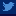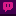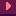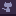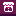Back A simple and generic 2D engine, part 2

In the previous article, I presented a simple, yet functional, 2D engine that you can use in any language (I used the Haxe language). It handles basic physics and level collisions.

But what about collisions between entities?

It may sound tricky, but it’s actually not that hard, if your game doesn’t require super high quality collisions.

## Demo

The following demo is not a platformer, but a top-down demo. That’s the same thing as a platformer, without gravity. That’s the engine used in Atomic Creep Spawner. This is what you will be able to do at the end of this tutorial.

Use arrow keys to move the yellow ball around.

Note : the “brutal” repositioning that occurs sometime is due to way the level collisions are handled. Instead of setting, say, xr to 0.3 if it’s lower than 0.3, you get better looking results adding a force to dx to repel the entity from the wall (and cap xr to 0.1 as a hard limit).

## Let’s start

Right now, let’s see what we have. Here is the pseudo code of the Entity class:

``````class Entity {
// Graphical object
public var sprite : flash.display.Sprite;

// Base coordinates
public var cx : Int;
public var cy : Int;
public var xr : Float;
public var yr : Float;

// Resulting coordinates
public var xx : Float;
public var yy : Float;

// Movements
public var dx : Float;
public var dy : Float;

public function new() {
//...
}

public function update() {
// X coord management (ie. movements + level collisions)
//...

// Y coord management (ie. movements + level collisions)
//...

// Graphical update
//...
}
}``````

## Detecting entities collisions

The idea here is, again, simplicity.
Each entity will have a radius which will define it’s “hitbox” for entity collisions. Note that, for the level collisions, we will still use the simple coordinate based code.

``public var radius : Float;``

Unless the precision of the collisions between entities is absolutely crucial in your gameplay (like in a physics based gameplay), simple circular collisions like this are more than enough.

Also, remember that this approach is only a basis: it’s minimal, so easy to maintain and expand.

In the following screenshot (source: arkadeo.com), the real collision areas are shown by the yellow circles. In a shoot em up, small hitboxes like these allow the player to actually brush past enemies (which is usually a satisfying feeling) :)

We can write a pretty simple collision detection method:

``````public inline function overlaps(e:Entity) {
// classic distance formula
var distSqr = (e.xx-xx)*(e.xx-xx) + (e.yy-yy)*(e.yy-yy);
return distSqr<=maxDist*maxDist;
// note: square root is not required this comparison.
}``````

Note that the method is inlined (ie. it’s content will be copied in place of its call by the Haxe compiler) because some target platforms, like Flash, have a small performance cost for function calls.

Ok! Now you can answer the question “does the hero collides with this other entity (like, say, an enemy or an item)”. Cool, we can already make a game out of it.

## Repel

In a game, many collisions between 2 entities result in the actual destruction of one (ex: picking up an item, getting hit by a bullet…). However, sometime, you need to put these entities in a situation where they don’t collide anymore (aka. repeling).

Let’s say we have 2 entities colliding: Blue and Yellow. We can say they are colliding because the distance between them is lower than the sum of their radiuses.

What we simply have to do is to slightly push Blue to the bottom left, and Yellow to the upper right. There are many approaches to do that :

1. add some repel force to the `dx,dy` values of each entity so they will bounce on each other.
2. recalculate the position of each Entity so their angle of incidence remains unchanged but they don’t overlap anymore
3. on each frame, slightly push their `xr,yr` away from the center of the collision without changing their current forces (dx,dy).
4. …a world of possibilities.

The choice depends on what you want to achieve, and the tolerance you actually have to how much they will overlap. For example, in solution 1, if two entities suddenly overlap (like Yellow falls with high velocity on Blue), they will really overlap for a few frames, before the repel force applies enough to separate them.

I usually use solution 1, the simplest (you know I like that). If it doesn’t fit my needs, I add into the mix other things like brutal repositioning (solution 2)…

Note: in the above example, the red line is the distance of overlapping. That’s a useful information if you want to calibrate the repel force based on how much the entities are actually overlapping. Or even choose to brutally reposition them if this overlap is really TOO much.

So!

The collision code will be placed in the `update`, before the X and Y management in our current source code. The reason: we usually want the level collisions to prevail on potential entity collision repeling (you probably don’t want your entities to get stuck into a wall). With repel force, this should not occur, but I don’t know what kind of horrible code my repel algorithm will evolve into, as the game evolves.

For each Entity, you need to check every other entities for collision.

THEORICAL WARNING: this can be really expensive if you have lots of entities in your game.
REALITY: usually no, you don’t have that much entities that collide and need to be repeled. Example: usually, bullets should not collide like this, they hit their target and simply die. Well, even if you do have many entities, their are lots of solutions to filter out the ones that have no chance to collide with the active one.

Like comparing their cx,cy coordinates.

``````for( e in ALL ) {
// Fast distance check
if( e!=this && Math.abs(cx-e.cx) <= 2 && Math.abs(cy-e.cy) <= 2 ) {
// Real distance check
var dist = Math.sqrt( (e.xx-xx)*(e.xx-xx) + (e.yy-yy)*(e.yy-yy) );
var ang = Math.atan2(e.yy-yy, e.xx-xx);
var force = 0.2;
dx -= Math.cos(ang) * repelPower * force;
dy -= Math.sin(ang) * repelPower * force;
e.dx += Math.cos(ang) * repelPower * force;
e.dy += Math.sin(ang) * repelPower * force;
}
}
}``````

The first IF is our basic “Do I really need to check this one? Is it even close enough?“.

Then we go with the real Square root distance thing. Then, if we actually have collision, we calculate their angle of incidence with the magic ATan2 formula.

We also compute a `repelPower` which is a factor (0 to 1) depending on how much the entities actually overlap.

We have an angle, we have “penetration factor”, we can add the scaled `force` to `dx` using the Cos of the angle, and to `dy` using the Sin.
The opposite (negative) is applied to the other entity.

That’s it.

1.:

Hi Sebastien,
Thanks for the tutorial!
I'm wondering when xx and yy are set? Preupdate?
Isn't there a chance for them to desynchronize from cx + xr.

What do you think about using an xx getter or setters (on cx, xr, or xx) to encapsulate this?

February 7, 2021 at 23:31
2.:

hi rly nice stuff.
I try to figure out a way to get rid or reduce the physic2d cost on Unity. And maybe it's worth a try to kick the Oncollision and filter like you do with a AABB(box collider)
Thx for sharing

November 21, 2020 at 11:22
3.:

Hey man, I know these tutorials are old, but they were super helpful when I got started, thanks for taking the time to write them. Your games are awesome! You are a huge inspiration!

May 13, 2019 at 06:53
4.:

Just a minor optimization: atan2, sin and cos can take many cpu cycles, depending on what you run it on. And you do not actually need the angle here, since

Math.cos(ang) is equal to (e.xx-xx)/dist
Math.sin(ang) is equal to (e.yy-yy)/dist

And I personally also find that easier to read, "split the force to x and y the same amount as the distance is split". But that might be a personal preference.

Also you could store e.xx-xx instead of calculating it thrice, but the compiler will probably do that for you.

But neither of these will probably be your performance bottleneck, so go with whatever reads best for you.

May 13, 2015 at 13:59
•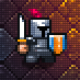:

Very interesting, thanks for the tip!

May 13, 2015 at 15:15
5.:

Nice !

May 17, 2013 at 21:47
6.:

Is there a time where the squares of the distance is less but the actual distance is not? It seems like you don’t need to call sqrt at all in the collision check. But I might be missing something. :-)

May 17, 2013 at 20:30
•:

Without square root, the calculation gives you: is the point (e.xx,e.yy) inside a SQUARE around (xx,yy)? With the square root, you get a little more precision: is the point (e.xx,e.yy) inside a CIRCLE around (xx,yy)? :)

May 17, 2013 at 20:41
•:

I think he might be right about not needing the sqrt.

var dist = ((e.xx-xx)*(e.xx-xx) + (e.yy-yy)*(e.yy-yy));
dist = Math.sqrt(dist2); //if you still need dist for later calcs

Thanks for all the info and tutorials. They are quite nice; I really like your style! Good job on your ludum dare entries as well, stunning!

You’ve inspired me to take a look at Haxe!
Keep up the great work!
:D
•